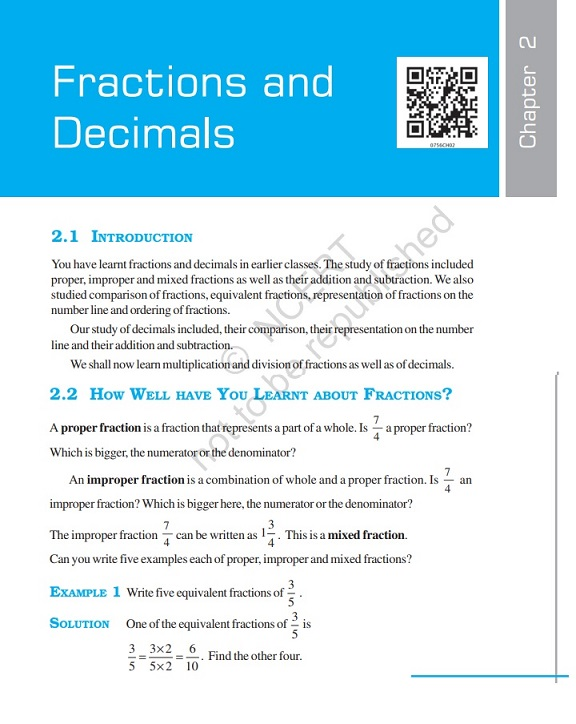Chapter 2 (Fractions and Decimals) of the NCERT Class 7 Mathematics Book (PDF) is available here for download as a PDF. Download and prepare for the CBSE Class 7 math exam.

Created on: Jul 14, 2021 14:17 IST

Fractions and Decimals – Chapter 2: Class 7 Maths NCERT Book (PDF)

Chapter 2 (Fractions and Decimals) of the NCERT Class 7 Mathematics Book (PDF) is available here for download as a PDF. Download and prepare for the CBSE Class 7 Mathematics Exam. This is one of the most important chapters of the CBSE Class 7 Maths NCERT textbook and many important questions are formulated from this chapter.

Fractions and Decimals – Chapter 2: Class 7 Maths NCERT Book (PDF): Download now

A Snapshot of the NCERT Class 7 Math Book (PDF) Chapter 2:Important topics in this chapter:

– Appropriate fraction

– Improper fraction

– Multiplication of fractions

– Multiplication of a fraction by a whole number

– Fraction as operator “of”

– Multiplication of a fraction by a fraction

– Division of fractions

– Division of the whole number by a fraction

– Reciprocal of a fraction

– Dividing a fraction by a whole number

– Division of a fraction by another fraction

– Multiplication of decimals

– Multiplication of decimals by 10, 100 and 1000

– Division of a decimal number by an integer

– Division of a decimal number by another decimal number

– Two fractions are multiplied by multiplying their numerators and denominators separately and writing the product in the form (product of numerators) / (product of denominators). For example, (2/3) x (5/7) = (2 Ã— 5) / (3 x 7) = 10/21

– A fraction acts as a ‘of’ operator. For example, (1/2) of 2 is (1/2) x 2 = 1

Fractions and Decimals – Chapter 2: Class 7 Maths NCERT Book (PDF): Download now

Share.